Mathematical Calculators

# Triangle Hypotenuse Calculator

Find out hypotenuse for all kinds of triangles easily with our free math calculator!

#### Triangle hypotenuse by one side and area

 ◦What is the hypotenuse of a triangle? ◦Why is the hypotenuse the longest side of the triangle? ◦How to calculate the hypotenuse of a triangle? ◦Good to know about Trigonometric functions ◦Classification of triangles based on the sides ◦Classification of triangles based on the angles ◦Fun facts about triangles

## What is the hypotenuse of a triangle?

The hypotenuse is the longest side of a triangle. It is also the side opposite from the right angle (90°).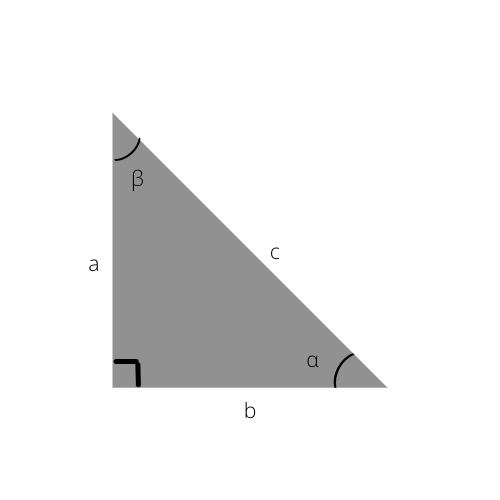The hypotenuse is c in this triangle.
You could also check out this Wikipedia article:
Hypotenuse - Wikipedia

## Why is the hypotenuse the longest side of the triangle?

After observing the picture above, and other right triangles, you will notice that the hypotenuse is always the longest side of all the right triangles. This is simply because it is located opposite of the biggest angle, the 90° angle.
this can also be proven mathematically by using the Pythagorean Theorem:
a² + b² = c²
a² > b² , a² > c²
a > b , a > c
As you see, the result of the operation above is that "a" (the hypotenuse) is bigger than the other two sides.

## How to calculate the hypotenuse of a triangle?

This can be done in 3 different ways, depending on the given information that can be a variation of the factors listed below:
a: opposite side
c: hypotenuse side
α: angle between the adjacent and the hypotenuse
β: angle between opposite and hypotenuse

### 1) Two right triangle legs

Formula: c = √(a² + b²) or c² = a² + b²
This formula is based on the Pythagorean theorem which can be simply utilised by taking a square root of the sum of squares of the adjacent and opposite.

### 2) Angle and one leg

Formula: c = a / sin(α) = b / sin(β)
You can also calculate the hypotenuse by utilising the law of sines, which is the basis of this formula.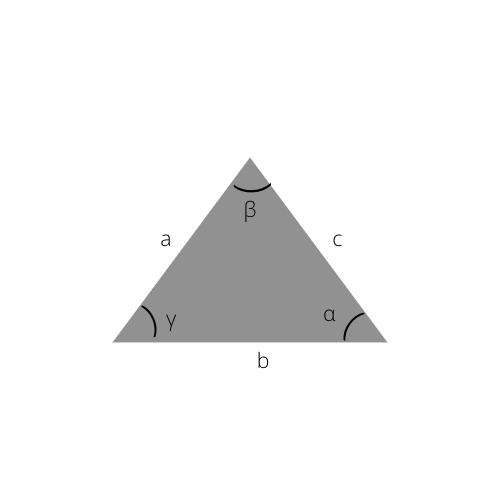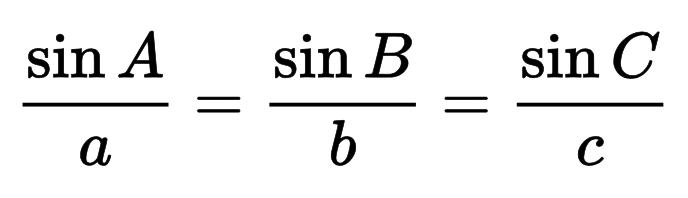The general law of sines

### 3) Area and one leg

Formula: c = √(a² + b²) = √(a² + (area _ 2 / a)²) = √((area _ 2 / b)² + b²)
This formula is based on the formula we use to calculate the area of a triangle (a \* b / 2). Compared to the other two it looks more complicated, however, it follows the same logic as the other two ways of calculating hypotenuses.

## Good to know about Trigonometric functions

If you're still keen on knowing more about the right triangle, check out these Trigonometric functions.sine - sin α = opposite / hypotenuse
cosine - cos α = adjacent / hypotenuse
tangent - tan α = opposite / adjacent
Knowing these, you can easily calculate the sides of the right triangle, or even determine the angles using the Trigonometric table below.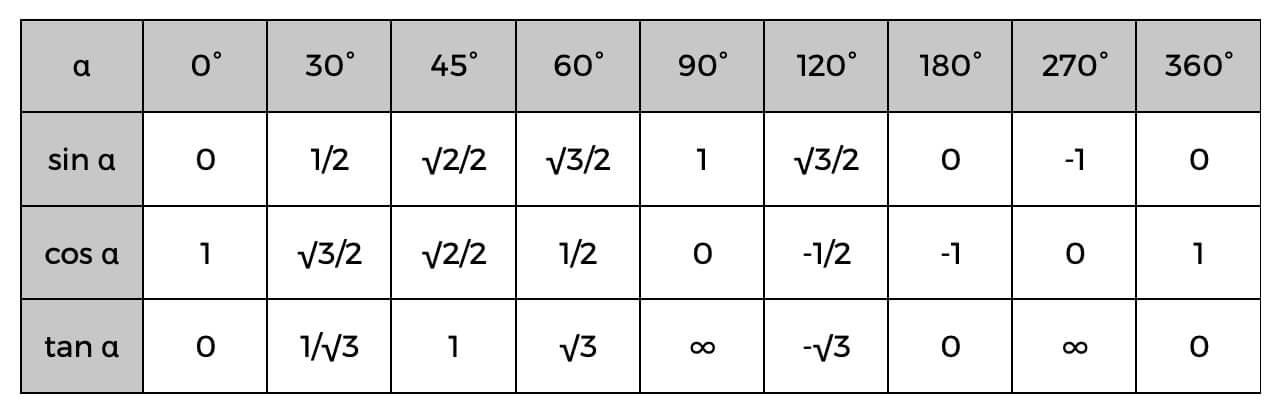An example of this can be that you already know the value of the hypotenuse and the adjacent; you can easily find the cosine of the angle, then check the table above to find the exact angle or just an estimation of what it could be. If the cosine of alpha (α) is 0.5, then we know that the angle is 60°.
You could also check out this Wikipedia article:
Trigonometric functions - Wikipedia

## Classification of triangles based on the sides

### 1) Equilateral

This triangle has three equal sides. This results in all the angles being 60°.
Visual example:Equilateral triangle

### 2) Isosceles

In this triangle only two sides are equal.
Visual example:Isosceles triangle

### 3) Scalene

None of the sides are equal in this triangle.
Visual example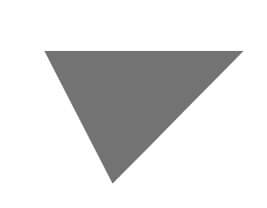Scalene triangle

## Classification of triangles based on the angles

### 1) Acute

All three angles in this triangle are smaller than 90°.
Visual example:Acute triangle
--

### 2) Right

This triangle has only one 90° angle, resulting in the other two being less than 90°.
Why?
α + β + γ = 180° & α = 90° → β + γ = 90° → β , γ < 90°
Visual example:Right triangle

### 3) Obtuse

This triangle has one angle that is greater than 90°.
Visual example:Obtuse triangle

### Fact 1:

If the triangle's inner-altitude is drawn, we get two right triangles in the original triangle.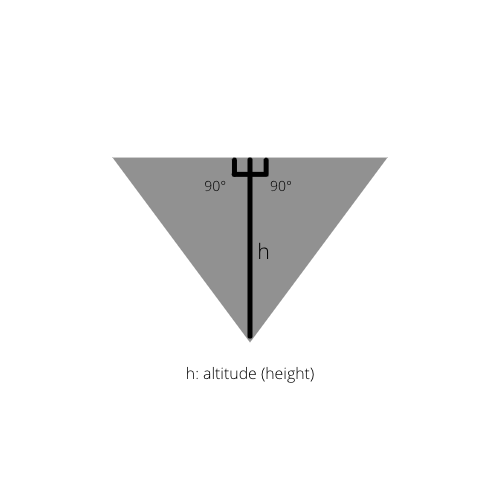### Fact 2:

As we know, the area of any triangle (A) is half of the height multiplied by the base (A = 1/2 _ b _ h). This formula can be written in a special way for the isosceles right triangle since its area is half of a square's area.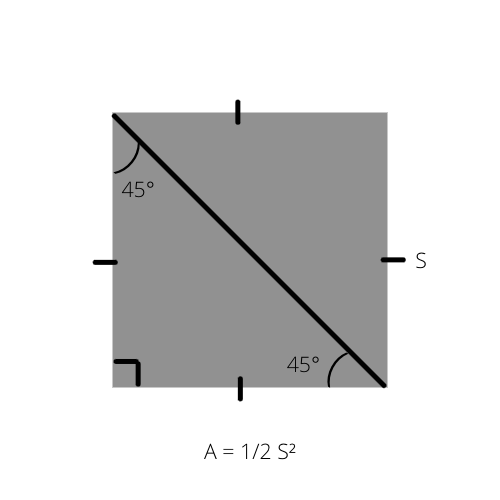A being the area of the triangle, and S the side of the square.

### Fact 3:

The sum of all three angles of a triangle is always 180°. This is true about all triangles.Article author
Parmis Kazemi
Parmis is a content creator who has a passion for writing and creating new things. She is also highly interested in tech and enjoys learning new things.

###### Triangle Hypotenuse Calculator English
Published: Wed Oct 27 2021
In category Mathematical calculators